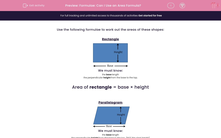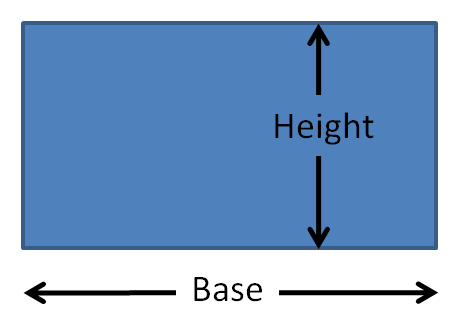# Formulae: Can I Use an Area Formula?

In this worksheet, students must recognise when it is possible to use formulae for the area of the given shapes.This content is premium and exclusive to EdPlace subscribers.Key stage:  KS 2

Curriculum topic:   Maths and Numerical Reasoning

Curriculum subtopic:   Perimeter and Area

Difficulty level:#### Worksheet Overview

Use the following formulae to work out the areas of these shapes:

RectangleWe must know:

• the base length
• the perpendicular height from the base to the top.

Area of rectangle = base × height

ParallelogramWe must know:

• the base length
• the perpendicular height from the base to the top. (NOT the slant height)

Area of parallelogram = base × height

TriangleWe must know:

• the base length
• the perpendicular height from the base to the apex. (NOT the slant heights)

Area of triangle = ½ base × height

or

Area of triangle = base × height ÷ 2

Example 1

Can an area formula be used to work out the area of this parallelogram, and if so, which one?This is a parallelogram.

We must know:

• the base length
• the perpendicular height from the base to the top. (NOT the slant height)

We know the base length and the slant height, but not the perpendicular height.

No, we cannot use the area formula.

Example 2

Can an area formula be used to work out the area of this triangle, and if so, which one?This is a triangle.

We must know:

• the base length
• the perpendicular height from the base to the top. (NOT the slant height)

If we turn our heads to the left, the base length is 12 cm and the perpendicular height is 6 cm.

We do not need to know the slant height of 10 cm to use the formula.

Yes, we can use the area formula:

Area of triangle = ½ base × height

### What is EdPlace?

We're your National Curriculum aligned online education content provider helping each child succeed in English, maths and science from year 1 to GCSE. With an EdPlace account you’ll be able to track and measure progress, helping each child achieve their best. We build confidence and attainment by personalising each child’s learning at a level that suits them.

Get started••••